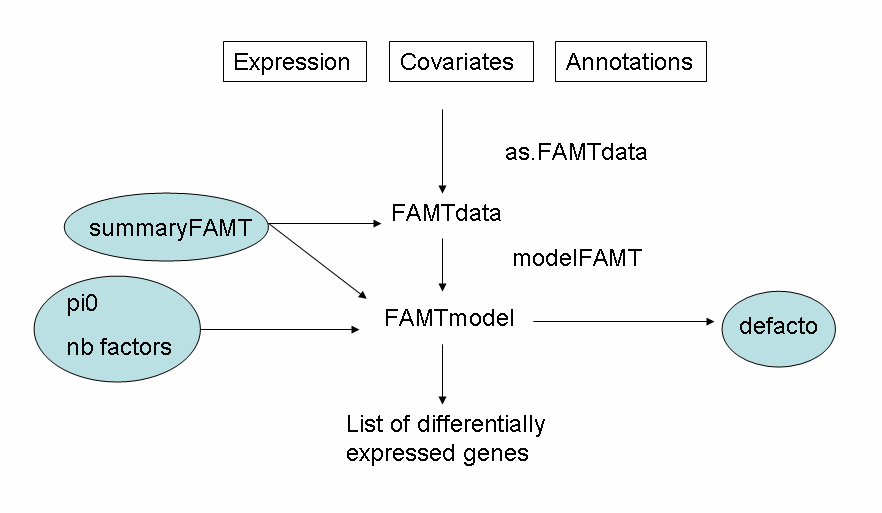## The package

The FAMT package is illustrated in Causeur et al. (2011) by two microarrays data analyses. The R functions, corresponding to the steps of the procedure, are detailled in the FAMT-manual. We briefly present the general structure of the package and its main functions (figure below).Figure 1. General structure of the FAMT package

The steps of the analysis correspond to core functions: as.FAMTdata to import data and create a single R list, modelFAMT to estimate the dependence kernel and adjust the data from heterogeneity components and defacto to relate the heterogeneity components to external information. Additional functions are proposed to summarize the results (summaryFAMT), and to optimize the procedure, such as the estimation procedure for the proportion of true null hypotheses (pi0FAMT).

The as.FAMTdata function creates a single R list from multi-sourced datasets:

• - a mandatory data-frame: the expression dataset with m rows (if m tests) and n columns (n is the sample size) containing the observations of the responses.
• - and two optional data frames: the covariates dataset with n rows and at least 2 columns, one giving the specification to match 'expression' and 'covariates' and the other one containing the observations of at least one covariate. The optional dataset, annotations, can be provided to help interpreting the factors: with m rows and at least one column to identify the variables (ID).

This function checks the consistency of the dataframes between them and creates the FAMTdata R object which is used in other functions of the package.

The modelFAMT function implements classical multiple testing procedure controlling the False Discovery Rate without any modeling for the dependence structure across the variables and the whole FAMT procedure.

The whole FAMT procedure is implemented with default options for the estimation of the proportion of true null hypotheses (pi0) and the number of factors. The number of factors considered in the model is chosen to reduce the variance of the number of false positives. Factor-adjusted test statistics are derived, as well as the corresponding p-values. The whole multiple testing procedure is provided in this single function, but you can also choose to apply the procedure step by step, using the functions:

• - nbfactors: Estimation of the optimal number of factors. The optimal number of factors of the FA model is estimated to minimize the variance of the number of false positives in multiple tests.
• - emfa: EM fitting of the Factor Analysis model.

The modelFAMT function creates a single R object which is used in other functions of the package.

The summaryFAMT function produces classical statistical summaries of FAMTdata. Moreover it provides the table of differentially expressed genes from the FAMTmodel and an estimation of pi0. The pi0FAMT function gives similar results of pi0 using alternative method. The defacto function provides diagnostic plots to interpret and describe the factors using external information either on genes or arrays.

## FAMT Method

The method proposed in this package takes into account the impact of dependence on the multiple testing procedures for high-throughput data. The common information shared by all the variables is modeled by a factor analysis structure. New test statistics for general linear contrasts are deduced, taking advantage of the common factor structure to reduce correlation and consequently the variance of error rates. This method improves the conditional FDR estimate and the overall performance of multiple testing procedure (decreasing the no-discovery proportion). The number of factors considered in the model is chosen to reduce the variance of the number of false discoveries. The model parameters are estimated using an EM algorithm. Factor-adjusted tests statistics are derived, as well as the corresponding p-values. The proportion of true null hypotheses (an important parameter when controlling the false discovery rate) is also estimated from the FAMT model.

The method captures the components of expression heterogeneity into factors. The common information shared by all the variables (i.e. gene expressions) is modeled by a factor analysis structure. Let Y(k)=(Y(1),Y(2),...,Y(n))' be a m-vector and x(k)=(x(1),...,x(p))' some explanatory variables. It is assumed that the conditional covariance matrix of the responses, given the explanatory variables, is represented by a factor analysis model:

Σ=ψ+BB'

where Ψ is a diagonal m x m matrix of uniqueness ψ2 and B is a q x m matrix of factor loadings. The diagonal elements ψ2 in Ψ are also refered to as the specific variances of the responses. BB' appears as the shared variance in the common factor structure.
The factor anaysis representation of the covariance is equivalent to the following mixed effects regression modeling of the data: for k=1,...,m

Y(k)0(k)+x'β(k)+b'kZ+ε(k)

where bk is the kth row of B, Z=(Z(1),..., Z(q)) are latent factors supposed to concentrate in a small dimension space the common information in the m responses, Z is normally distributed with expectation 0 and variance Iq and ε=(ε(1), ...,ε(m))' is a normally distributed m-vector, independant of Z, with mean 0 and variance-covariance Ψ.

An EM algorithm is used to estimate Ψ, B and Z. The number of factors is chosen so that the variance of the number of false discoveries is minimized. Factor-adjusted tests statistics are obtained by correction of the classical t-tests from the effect of the common factors. Friguet et al. (2009) show that the resulting tests statistics are asymptotically uncorrelated, which improves the overall power of the multiple testing procedure.